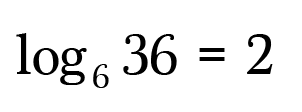# Understanding Logarithms and Roots

Logs & roots — no, I’m not talking about trees. I’m talking about the mathematical kind. I bet you’re thinking,

“Roots, okay. But logarithms? Isn’t that an algebra 2 topic?!”

Yep, it is! But who says we can’t learn it right now? Why save for later what can be learned today? Carpe diem, am I right? But first, let’s do a quick review. Recall this diagram on exponents from lesson two?

The number we multiply with itself is called the base. The number of times we multiply it with itself is called the power or exponent.

Here are a few examples of exponents to refresh your memory.

We’d like to find an operation to get back the base number from the solution of an exponential equation. That’s where roots come in.

### Square Roots, Cube Roots and More

Suppose instead of finding the square of 9, which is 81, we wanted to find out what number multiplied with itself equals 81.

In other words, what is the square root of 81?

This equals 9 because nine squared is eighty-one.

We can take the square root of any non-negative number, but only perfect square numbers yield whole number results. So familiarize yourself with those first. Here are a few to get you started:

Now for some terminology.

The root index is optional on square roots. Square roots are often written:

The index is only necessary to distinguish between higher indexed roots, such as cube roots, fourth roots, fifth roots, etc.

Cube roots ask you to find the number that when multiplied with itself three times yields the radicand, like these:

Fourth roots ask you to find the number that when multiplied with itself four times yields the radicand.

Fifth roots ask you to find the number that when multiplied with itself five times yields the radicand.

Again, you can take any root of any non-negative number (and in certain cases of negative numbers as well), but for many numbers you’ll need a calculator since the answers are irrational. The examples above are the “nice cases” that come up frequently!

### Logarithms

What if we wanted to solve for the exponent in an exponential equation? In other words, we want to reverse the exponentiation. For example, what is the solution to this problem?

Since we’ve memorized the common powers and roots, we easily identify the solution as 2 since 6 to the power of 2 is 36.

Writing a question mark in the equation isn’t formal mathematics, instead we’ll write the above expression using logarithm notation, or log for short.Read: “the log, base six, of thirty-six is 2.”

The terminology:

Another way to look at this is to ask,

How many sixes need to be multiplied together to get 36?

A logarithm solves for the number of repeated multiplications. Simple as that. Here are a few more examples.

Thanks for reading!

#### ❤ STAY CONNECTED ❤

Stay up-to-date with everything Math Hacks is up to!